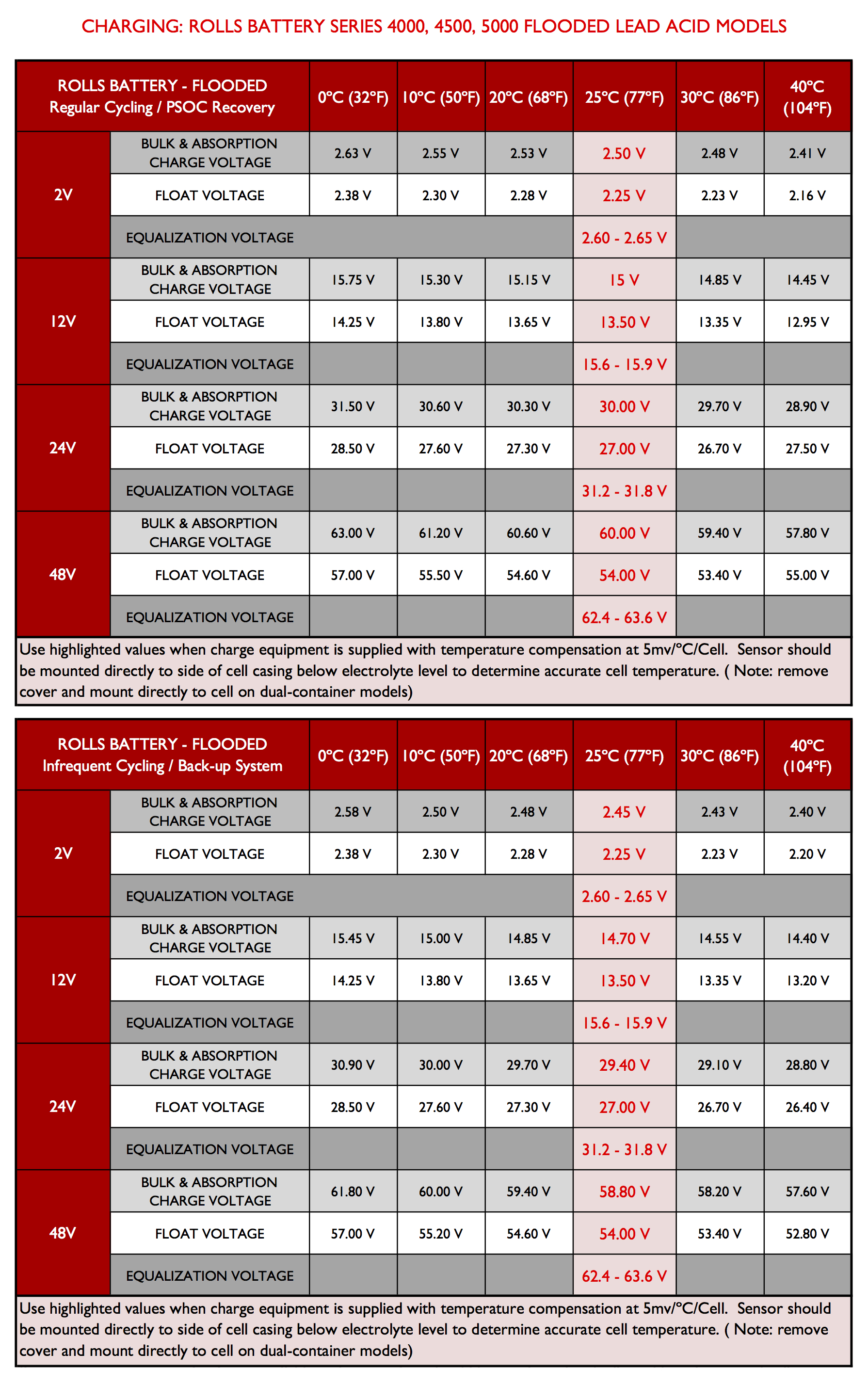State of Charge and Charging

The truest measure of a battery's state of charge is the SPECIFIC GRAVITY of the battery acid. The following shows the approximate state of charge at various specific gravities at 77ºF / 25ºC.

 Charged Specific Gravity 100% 1.255-1.275 75% 1.215-1.235 50% 1.180-1.200 25% 1.155-1.165 0% 1.110-1.130

Hydrometers can be difficult to use and at best accurate to +/-0.005 points. Voltage can be used to estimate state of charge but caution must be taken when interpreting voltage readings.

All Amphere Hour capacities based on specific gravities of 1.280. Reduce capacities 5% for 1.265 specific gravity and 10% for specific gravities of 1.250

We recommend a three step charging procedure. Recommended voltage settings are as follows:To calculate the correct settings for another battery bank voltage divide the total nominal voltage by two and use this number as a multiplier. For example a 18V system, 18/ 2 = 9, equalization preferred = 9 x 2.58 = 23.2 V

NOTE: The ideal float voltage is the lowest voltage setting that will maintain the battery at full charge. The higher the voltage the more water the cell will consume. The minimum equalization voltage is highly recommended unless it is suspected a sulfation problem exists and a corrective equalization is required.

Rebulk Voltage:  This setting determines the battery bank voltage may drop between a Float and Bulk charge.  It may be set at personal preference.  For assistance and recommendation, please refer to the charge controller manufacturer for instruction and detailed information on this setting.

Absorption Time:

The most important part of the charge cycle is the absorption charge. Since the bulk charge only recharges the battery bank to an 80% level, the absorption charge completes the charging cycle. Most chargers on the market have a timer that allows the user to adjust the duration for the required time to return the battery to full charge. In order to set the correct time, a simple calculation is required. With the help of the 20 AH capacity, you can figure out the remaining charge required for the battery bank using the following equation:

t = 0.42 x (C/I)

Where:

t = Absorption Charge Time (Hours)

C = 20 hr Rated Capacity (AH)                [ex: 2 strings x S6 L16-HC models (428 AH)  = 856 AH rated capacity]

I = Charging Current (Amps)                  [charger output min 5% up to max 10% of 20 hr rate]

EXAMPLE:

2 strings of S6 L16-HC batteries

20 hr rate = 428 AH x (2 strings) = 856 AH

I =10% of 856 AH = 85 amps    OR      If charger is only 60 amps max then 60 is used

T = 0.42 x 856/85 = 4.2 hrs
OR      T = 0.42 x 856/60 = 6 hrs

We recommend a charge current of 10% of the 20 hr rate for both Bulk & Absorption settings.  [5% min, 20% max]